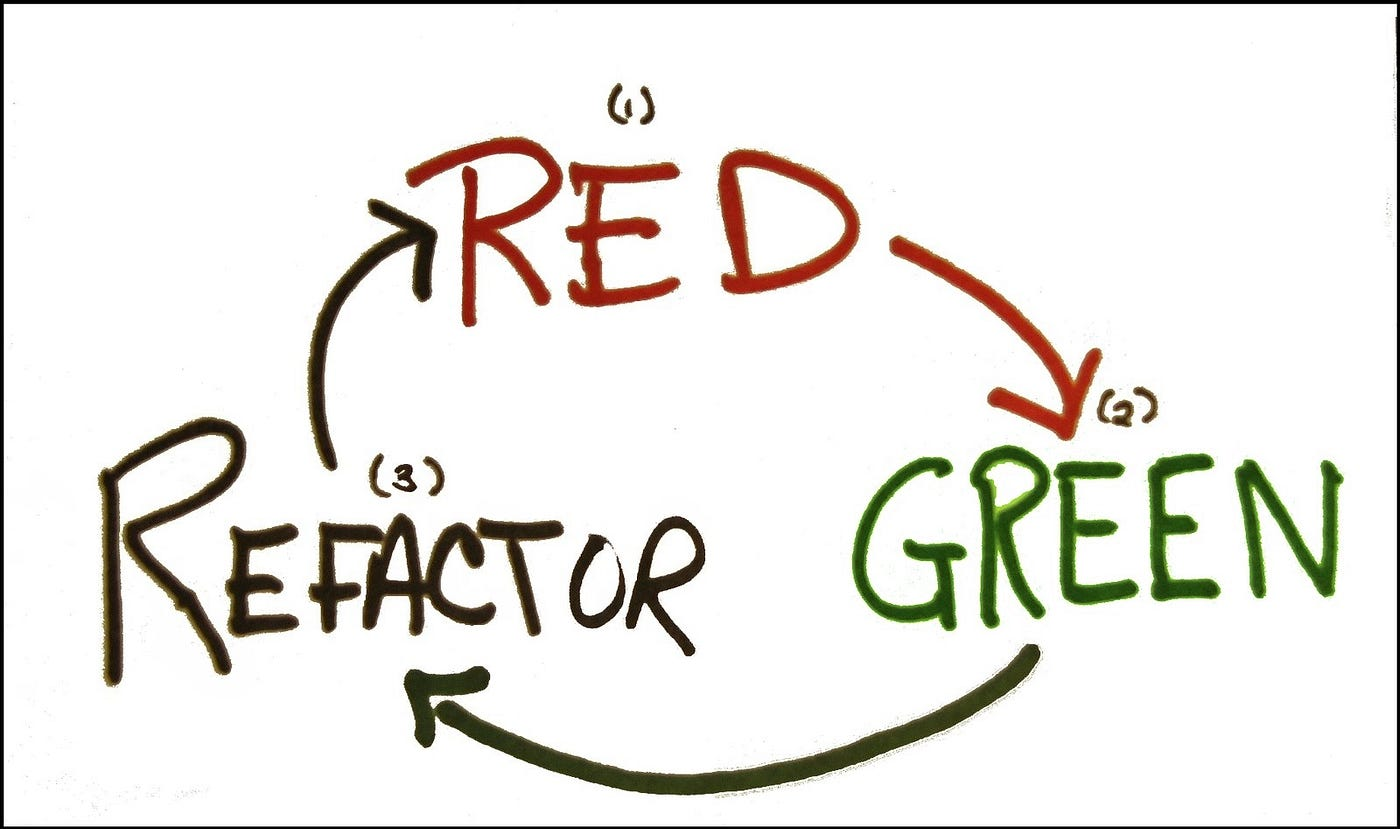# The 3-step process of TDD

1. Write a test, watch it fail
2. Write code to make the test pass
3. Refactor the code3-step process of TDD — Image credit dywl

# How to do TDD

## Project requirement

`// Addition in javascript2 + 2 = 4;2 + “2” = 22; // oh oh!`

## Getting started

`“scripts”: { “test”: “mocha”}`

## Write a test that fails

`const chai = require(‘chai’);describe(‘#add’, () => { it(‘should add two numbers correctly’, () => { expect(add(2, 2)).to.equal(4); });});`

## Write code to pass the test

`function add (a, b) { return a + b;}module.exports = add;`
`const chai = require(‘chai’);const add = require(‘../addition’);describe(‘#add’, () => { it(‘should add two numbers together correctly’, () => {    expect(add(2, 2)).to.equal(4); }); it(‘should throw error for invalid argument’, () => {    expect(() => add(‘4’, 2)).to.throw(‘Invalid argument’); });});`
`function add (a, b) { if (typeof a !== ‘number’ || typeof b !== ‘number’) throw new TypeError(‘invalid argument’);  return a + b;}module.exports = add;`